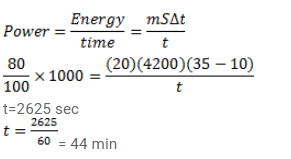# Calculate the time required to heat 20kgQuestion:

Calculate the time required to heat $20 \mathrm{~kg}$ of water from $10^{\circ} \mathrm{C}$ to $35^{\circ} \mathrm{C}$ using an immersion heater rated $1000 \mathrm{~W}$. Assume that $80 \%$ of the power input is used to heat the water. Specific heat capacity of water= $4200 \mathrm{~J} / \mathrm{kg}^{-\mathrm{K}}$

Solution: